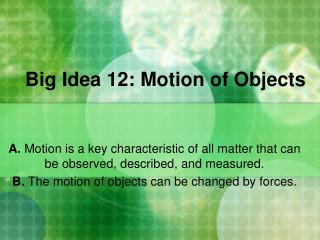DownloadDownload PresentationBig Idea 12: Motion of Objects

# Big Idea 12: Motion of Objects

Download Presentation## Big Idea 12: Motion of Objects

- - - - - - - - - - - - - - - - - - - - - - - - - - - E N D - - - - - - - - - - - - - - - - - - - - - - - - - - -
##### Presentation Transcript

1. Big Idea 12: Motion of Objects A. Motion is a key characteristic of all matter that can be observed, described, and measured. B. The motion of objects can be changed by forces.

2. Benchmark Number & Descriptor • SC.6.P.12.1 • Measure and graph distance versus time for an object moving at a constant speed. Interpret this relationship.

3. MOTION I know the car moved because they are in different locations based on the tree (reference point). • Motion is the movement of an object. • When identifying motion, you must use a reference point. • An object that appears to stay in one place • To describe the motion of an object, you would describe how the object’s direction, distance, or both, changed relative to the reference point.

4. SPEED • Rate of change of position • Calculation: • Speed = Distance Time

5. CONSTANT SPEED • The speed of the moving object is always the same no matter the location. • When graphing constant speed: • Distance on y-axis • Time on x-axis • Constant speed is shown as a straight line. • When in constant motion, the slope of the line does not change.

6. Graphing Constant Speed The slope of this line does not change. The distance on this graph stays the same Speed = d/t = 0/10 = 0 m/min The object is not moving. The slope of the line does not change. The change in distance for every minute on this graph is constant. Speed = d/t Speed = 2 m/min

7. Knowledge Check • How can you identify if an object is in motion? • 2. What is the definition and formula of speed? • 3. By looking at a graph, how can you tell if it is representing constant speed?

8. Knowledge Check • How can you identify if an object is in motion? • Find a permanent reference point. Compare before and after movements to the reference point. If the RP is in a different location from start to finish, the object has moved. • 2. What is the definition and formula of speed? • Rate of change of position • Speed = Distance/Time • 3. By looking at a graph, how can you tell if it is representing constant speed? • The slope of the line does not change at any point.